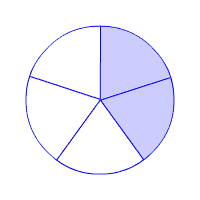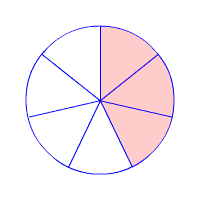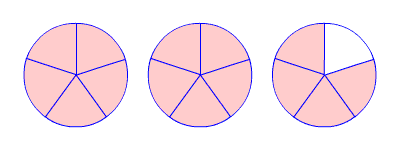# Fractions

A fraction is a way of expressing division.

$\frac{a}{b}$ means $a÷b$ .

This notation can be used to represent numbers which are not whole numbers .

The number below the bar is called the denominator .  It tells the number of equal parts into which the whole has been divided.

The number above the bar is called the numerator.   It tells how many of the equal parts are being considered.

Example 1:

$\frac{2}{5}$ means $2÷5$

The whole has been divided into $5$ parts and $2$ are being considered.

The fraction $\frac{2}{5}$ can be represented by a shape divided into $5$ pieces of equal size, with $2$ of them shaded.A proper fraction is a fraction whose numerator is less than its denominator.  If the numerator is greater than the denominator, then it is an improper fraction .

Example 2:

$\frac{3}{7}$ is a proper fraction . It can be represented by a shape divided into $7$ pieces of equal size, with $3$ of them shaded.$\frac{14}{5}$ is an improper fracti on . It is greater than $1$ , so to draw it, you'll need more than $1$ shape. In fact it needs $3$ equal shapes, each divided into $5$ pieces of equal size, and $14$ of them shaded.A number which consists of a whole number plus a fraction is a mixed number .   Mixed numbers can be written as an improper fraction and an improper fraction can be written as a mixed number. The improper fraction above can be written as $2\frac{4}{5}$ .

Example 3:

Write $7\frac{2}{5}$ as an improper fraction.

$7\frac{2}{5}=\frac{7}{1}+\frac{2}{5}$

$=\frac{7\text{\hspace{0.17em}}\cdot \text{\hspace{0.17em}}5}{1\text{\hspace{0.17em}}\cdot \text{\hspace{0.17em}}5}+\frac{2}{5}$

$=\frac{35}{5}+\frac{2}{5}$

$=\frac{37}{5}$

Example 4:

Write $\frac{11}{7}$ as a mixed number in simple form.

Therefore, $\frac{11}{7}=1\frac{4}{7}$ .

A fraction is in lowest terms when the numerator and denominator have no common factor other than $1$ .  To write a fraction in lowest terms, divide the numerator and denominator by the greatest common factor .

Example 5:

Write $\frac{45}{75}$ in lowest terms.

$45$ and $75$ have a common factor of $15$ .

$\frac{45}{75}=\frac{45\text{\hspace{0.17em}}÷\text{\hspace{0.17em}}15}{75\text{\hspace{0.17em}}÷\text{\hspace{0.17em}}15}=\frac{3}{5}$